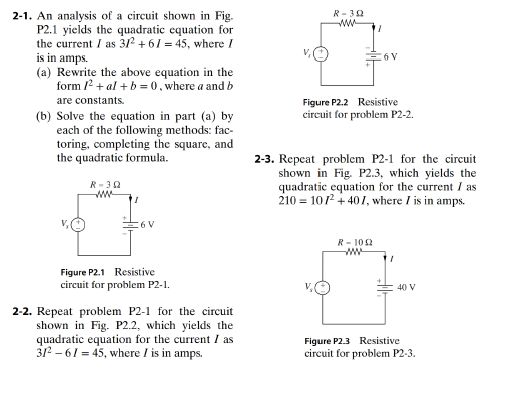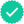#### Videos

Textbook QuestionChapter 2, Problem 1P

An analysis of a circuit shown in Fig. P2.1 yields the quadratic equation for the current I as 3 I 2 6 I = 45 , where I is in amps.

(a) Rewrite the above equation in the form I 2 + a I + b = 0 , where a and b are constants.

(b) Solve the equation in part (a) by each of the following methods: factoring, compacting the square, and the quadratic formula.Expert SolutionTo determine

(a)

To rewrite the given current relation in the form of I2+aI+b=0.

The required equation of current in the form of I2+aI+b=0 is

I2+2I+(15)=0

### Explanation of Solution

Given:

The current relation is given as

3I2+6I=45. ...... (1)

Calculation:

Divide by 3 on both sides in the equation (1)

I2+2I=15

Rearrange above equation.

I2+2I15=0

The required equation of current in the form of I2+aI+b=0 is

I2+2I+(15)=0

Conclusion:

Thus, the required equation of current in the form of I2+aI+b=0 is

I2+2I+(15)=0

Expert SolutionTo determine

(b)

Solve the equation I2+2I15=0 by factoring, completing the square, and the quadratic formula.

The equation is solved by factoring, completing the square, and the quadratic and the roots of the equation are 3 and 5.

### Explanation of Solution

Concept Used:

The roots of the quadratic equation can be determined by the quadratic formula given as-

I=b±b24ac2a ...... (2)

Calculation:

Substitute 2 for b, 1 for a and 15 for c in equation (2)

I=2± ( 2 ) 2 4( 1 )( 15 )2(1)=2±82

This equation gives two values of current

I=3 amps and I=5 amps

For completing square method,

Rearrange equation (1)

I2+2I=15

Add 1 on both side of the above equation.

I2+2I+1=15+1(I+1)2=16

Rearrange the above equation.

(I+1)216=0(I+1)2(4)2=0(I+14)(I+1+4)=0(I3)(I+5)=0

The value of current I are 3 amps and 5 amps

For factoring method,

Rearrange equation (1)

I2+(53)I15=0I2+5I3I15=0I(I+5)3(I+5)=0(I+5)(I3)=0

The value of current I are 3 amps and 5 amps

### Want to see more full solutions like this?

Subscribe now to access step-by-step solutions to millions of textbook problems written by subject matter experts!
07:34

Find more solutions based on key concepts
Knowledge BoosterRecommended textbooks for you
•Algebra & Trigonometry with Analytic Geometry
Algebra
ISBN:9781133382119
Author:Swokowski
Publisher:Cengage
•Algebra & Trigonometry with Analytic Geometry
Algebra
ISBN:9781133382119
Author:Swokowski
Publisher:Cengage